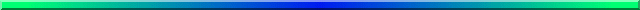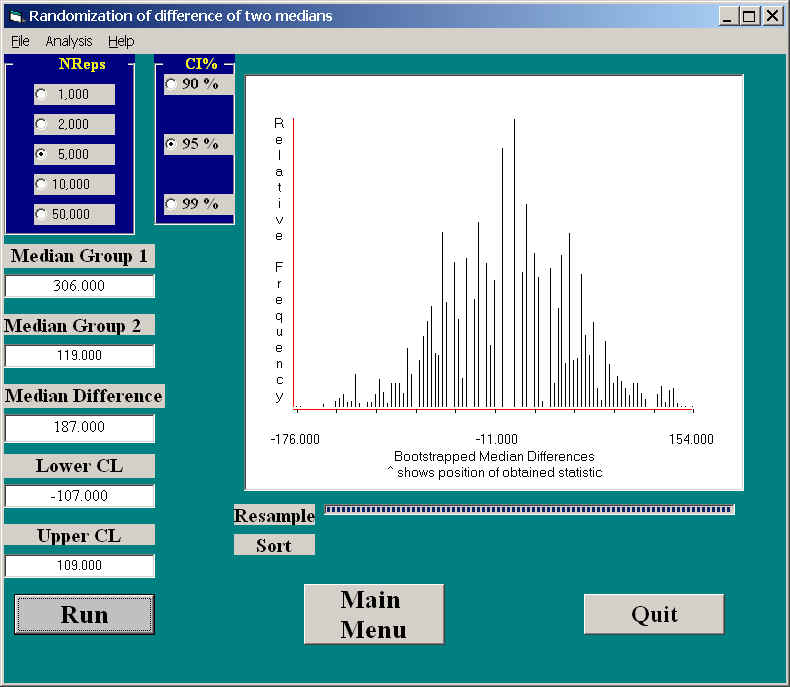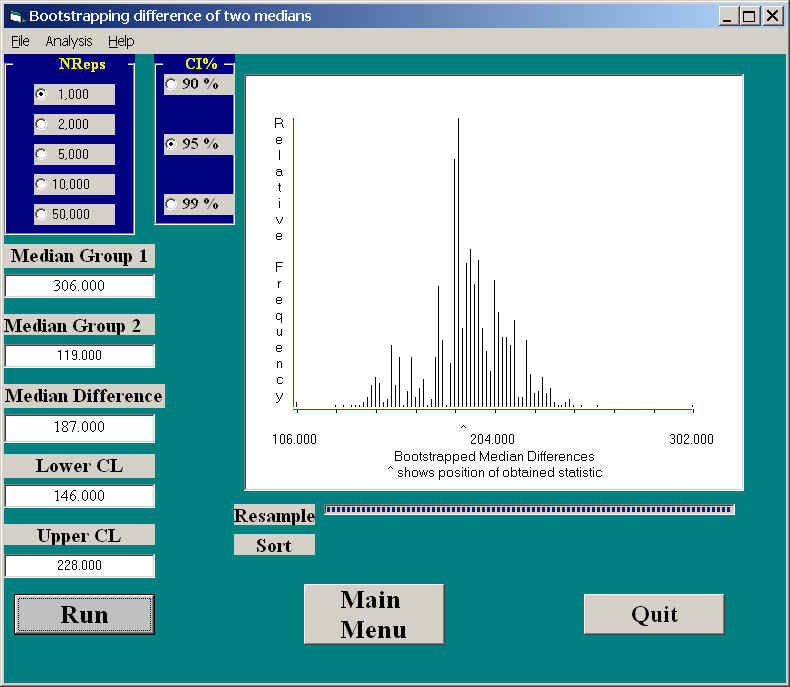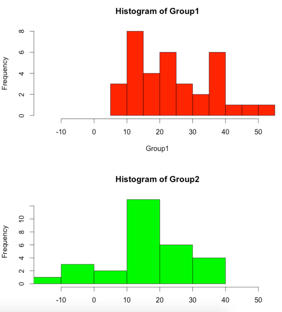# Randomization for Comparing Two MediansSuperficially, a test on medians is similar to a test on means. But there are fundamental differences. With the two previous randomization tests (two independent samples and two paired samples), we used the t computed between the means of two groups to measure differences. We don't have a t that we can use with medians, because we don't have a useful way of calculating the standard error, which is needed to compute a t.

One alternative to this might have been to take the obtained median difference as my statistic, and to count the number of randomizations that obtained a median difference as great as the one in our obtained data. That was not the approach that I took when I wrote the program included in Resampling.exe, although I may go back to that in the near future.

What I did was actually more in line with what we will do with bootstrapping. I calculate confidence limits on the median difference under the null hypothesis, and will then rejected the null hypothesis if the obtained difference in medians falls outside these limits. The advantage of this approach is that it gives us confidence limits, which are nice to have. On the other hand, the limits that it produces are probably not the ones that really interest us. These are the limits we would obtain when the null hypothesis is true, they are not limits on the true difference between medians. In fact, I am not sure how we would get limits on the true difference between medians with a randomization test. The disadvantage of the approach that I have taken is that it will show that you can reject the null if the obtained difference falls outside the interval, but it will not give you a p value on the probability of such a difference under the null.

As I say, I may change the approach. But until I do, I need to describe the one I used.

I will use the same example that I will use when comparing two medians with bootstrapping. This is a study by Merrell on maze performance of mice raised in the daily presence of music by Mozart or Anthrax. The data are available at Merrell.dat.

I used Merrell's data, which recorded the times each mouse took to navigate a maze. In the Mozart condition the median was 119 seconds, while in the Anthrax condition the median was 306 seconds. That is a difference of 187 seconds, which is approximately 3 minutes.

I created 5000 randomizations of the 24 + 24 = 48 observations. For each permutation the first 24 scores were assigned to the Mozart condition, and the last 24 observations to the Anthrax condition. I then calculated the median under each condition, and then the median difference. Across 5000 resamples, this gave me the sampling distribution of the difference between two medians, from which I calculated the limits of the confidence interval by what Efron calls the "percentile method." This method takes the lower and upper limits and the 2.5% and 97.5% points of the sampling distribution. As I explain elsewhere, I am not particularly comfortable with this approach, but it is a standard approach and, when the distribution is symmetric, as this one usually is, it produces nearly the same limits as other approaches.

The results of this approach can be seen in the following figure.As you can see, the 95% confidence interval on the difference in medians is -107 to +109. You would expect the interval to be nearly symmetrical about 0, and it is. You can also see the obtained median difference was 187, which clearly falls outside the interval. Thus we can reject the null hypothesis, and conclude that the median response time of the Anthrax condition is significantly greater than the median response time of the Mozart condition.

Notice that this sampling distribution is noticeably more sparse than the distributions we have seen for means. That is because of the limited possibilities for a median. It must either be one of the values in the original sample, or half way between two original values.

It is useful to compare this result against the one we will find when we use the bootstrapping approach. The two will be visibly different because the approach is different. With bootstrapping, we are able to set the confidence interval on the true difference in medians, whereas with randomization we are forced to set the confidence limits on the difference under the null. From the above figure I can tell you that my 95% confidence interval on the median when the null is true, is -107 to 109. (Actually, that is a pretty strange sounding statement, and I need to think a lot about it.) Because 187 is not included in that interval, we can reject the null. On the other hand, from the figure below I can tell you that the 95% confidence interval on the true difference between medians is 146 to 228. Because this interval does not include 0, we can also reject the null. Notice how different these two approaches are.# Using R as Alternative Software

Again we wioll have a fairly long section of code, but that stems largely from the fact that I am avoiding shortcuts and laying all of the code out to make it easier to follow.

```

#  Randomization test on two medians
#  Data from study by Adams et al. (Methods8, tab7.5)

set.seed(1086)
dat\$Group <- factor(dat\$Group)
n1 <- length(dat\$Arousal[dat\$Group == 1])
n2 <- length(dat\$Arousal[dat\$Group == 2])
N <- n1 + n2
Group1 <-  dat\$Arousal[dat\$Group == 1]
Group2 <-  dat\$Arousal[dat\$Group == 2]

md1 <- median(Group1, na.rm = T)
md2 <- median(Group2, na.rm = T )

par(mfrow = c(2,1))
hist(Group1, col = "red", xlim = c(min(dat\$Arousal), max(dat\$Arousal)) )
hist(Group2, col = "green", xlim = c(min(dat\$Arousal), max(dat\$Arousal)))obt.med.diff <- md1 - md2
cat("The obtained median difference is ", '\n', obt.med.diff, '\n')
_______________
The obtained median difference is
2.7
_______________
nreps = 5000
set.seed(1086)
samp.med.diff <- numeric(nreps)
for (i in 1:nreps) {
resamp.data <- sample(dat\$Arousal, length(dat\$Arousal), replace = FALSE)
Grp1 <-  resamp.data[1:n1]
Grp2 <-  resamp.data[(n1+1):N]
md1 <- median(Grp1, na.rm = T)
md2 <- median(Grp2, na.rm = T )
samp.med.diff[i] <- md1 - md2
}
obt.med.diff <- abs(obt.med.diff)
pUpper <- length(samp.med.diff[samp.med.diff >= obt.med.diff]) /nreps
pLower <- length(samp.med.diff[samp.med.diff <= (-1)*obt.med.diff])/nreps
pExtreme <- pLower + pUpper
cat("The two tailed probablility under the null is = ",'\n',pExtreme, '\n')

# Confidence limits
CIupper <- quantile(samp.med.diff,.975)
CIlower <- quantile(samp.med.diff,.025)
cat("The 95% confidence limits are = ", '\n',CIlower, " and ", CIupper, '\n')
#Output
#cat("The two tailed probablility under the null is =
# 0.2904
#Note the dramatic difference with the standard t test (Below).
#SPSS gives similar results for Wilcoxon and t tests, so it
#is probably not an error.
# Wilcoxon gives p = .315, t gives p = .015
#(t.test(Arousal~Group))     Remove # to get output

#####  Verifying using coin library  (COnditional INference library)
library(coin)
median_test(dat\$Arousal ~ dat\$Group, distribution = exact())
_______________
# median_test(Arousal ~ Group, distribution = exact())
#
#        Exact Median Test
#
#data:  Arousal by Group (1, 2)
#Z = -1.2457, p-value = 0.3152
#alternative hypothesis: true mu is not equal to 0

#t.test(Arousal~Group)
#
#        Welch Two Sample t-test
#
#data:  Arousal by Group
#t = 2.4917, df = 60.495, p-value = 0.01547
#alternative hypothesis: true difference in means is not equal to 0
#95 percent confidence interval:
#  1.47935 13.51375
#sample estimates:
#mean in group 1 mean in group 2
#       24.00000        16.50345

_______________

# This could be expanded to multiple groups, but I have not yet thought what
# a good test statistic would be. I would suggest trying the test
# statistic (H) for the Kruskal-Wallis test, but use the raw scores instead
# of the ranks. I haven't tried it, so I make no promises.dch: